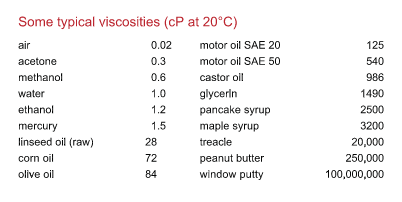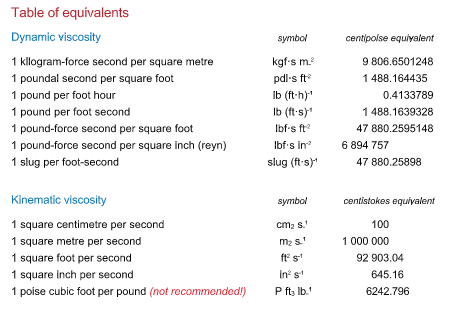# Units of Viscosity

Dynamic viscosity

Poise (symbol: P)

Named after the French physician Jean Louis Marie Poiseuille (1799-1869), this is the cgs unit of viscosity, equivalent to dyne-second per square centimetre. It is the viscosity of a fluid in which a tangential force of 1 dyne per square centimetre maintains a difference in velocity of 1 centimetre per second between two parallel planes 1 centimetre apart.

Even in relation to high-viscosity fluids, this unit is most usually encountered as the centipoise (cP), which is 0.01 poise. Many everyday fluids have viscosities between 0.5 and 1000 cP (see table).

Pascal-second (symbol: Pa·s)

This is the SI unit of viscosity, equivalent to newton-second per square metre (N·s m-2). It is sometimes referred to as the “poiseuille” (symbol Pl).

One poise is exactly 0.1 Pa·s. One poiseuille is 10 poise or 1000 cP, while 1 cP = 1 mPa·s (one millipascal-second).

Kinematic viscosity

Stokes (symbol: St)

This is the cgs unit, equivalent to square centimetre per second. One stokes is equal to the viscosity in poise divided by the density of the fluid in g cm–3. It is most usually encountered as the centistokes (cSt) (= 0.01 stokes).

Saybolt Seconds Universal (SSU)

This is the time for 60 ml of fluid to flow through the calibrated orifice of a Saybolt Universal viscometer at a specifi ed temperature, as prescribed by test method ASTM D 88. For higher

Degree Engler

This is the ratio of the time of fl ow of 200 ml of fl uid to the time of fl ow of 200 ml of water at the same temperature in a standardized Engler viscosity meter.viscosities, SSF (Saybolt Seconds Furol) is used. “Furol” comes from “fuel and road oil”.This technical brief was supplied by Hydramotion.

Updated

11 out of 19 found this helpful

Have more questions? Submit a request

<% if (previousArticle || nextArticle) { %>
<% if (previousArticle) { %>
<% if (previousTitle) { %>

#### <%= previousTitle %>

<% } %> <%= previousArticle.title %>
<% } %> <% if (nextArticle) { %>
<% if (nextTitle) { %>

#### <%= nextTitle %>

<% } %> <%= nextArticle.title %>
<% } %>
<% } %>
<% if (allItems.length > 1) { %>